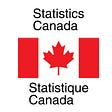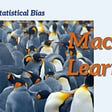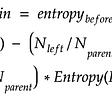# Why is scaling required in KNN and K-Means?

KNN and K-Means are one of the most commonly and widely used machine learning algorithms. KNN is a supervised learning algorithm and can be used to solve both classification as well as regression problems. K-Means, on the other hand, is an unsupervised learning algorithm which is widely used to cluster data into different groups.

One thing which is common in both these algorithms is that both KNN and K-Means are distance based algorithms. KNN chooses the k closest neighbors and then based on these neighbors, assigns a class (for classification problems) or predicts a value (for regression problems) for a new observation. K-Means clusters the similar points together. The similarity here is defined by the distance between the points. Lesser the distance between the points, more is the similarity and vice versa.

# Why do we need to scale the data?

All such distance based algorithms are affected by the scale of the variables. Consider your data has an age variable which tells about the age of a person in years and an income variable which tells the monthly income of the person in rupees:

Here the Age of the person ranges from 25 to 40 whereas the income variable ranges from 50,000 to 110,000. Let’s now try to find the similarity between observation 1 and 2. The most common way is to calculate the Euclidean distance and remember that smaller this distance closer will be the points and hence they will be more similar to each other. Just to recall, Euclidean distance is given by:

Here,

n = number of variables

p1,p2,p3,… = features of first point

q1,q2,q3,… = features of second point

The Euclidean distance between observation 1 and 2 will be given as:

Euclidean Distance = [(100000–80000)^2 + (30–25)^2]^(1/2)

which will come out to be around 20000.000625. It can be noted here that the high magnitude of income affected the distance between the two points. This will impact the performance of all distance based model as it will give higher weightage to variables which have higher magnitude (income in this case).

We do not want our algorithm to be affected by the magnitude of these variables. The algorithm should not be biased towards variables with higher magnitude. To overcome this problem, we can bring down all the variables to the same scale. One of the most common technique to do so is normalization where we calculate the mean and standard deviation of the variable. Then for each observation, we subtract the mean and then divide by the standard deviation of that variable:

Apart from normalization, there are other methods too to bring down all the variables to the same scale. For example: Min-Max Scaling. Here the scaling is done using the following formula:

For now, we will be focusing on normalization. You can try min-max scaling as well. Let’s see how normalization can bring down these variables to same scale and hence improve the performance of these distance based algorithms. If we normalize the above data, it will look like:

Let’s again calculate the Euclidean distance between observation 1 and 2:

Euclidean Distance = [(0.608+0.260)^2 + (-0.447+1.192)^2]^(1/2)

This time the distance is around 1.1438. We can clearly see that the distance is not biased towards the income variable. It is now giving similar weightage to both the variables. Hence, it is always advisable to bring all the features to the same scale for applying distance based algorithms like KNN or K-Means.

--

--

--

## More from Analytics Vidhya

Analytics Vidhya is a community of Analytics and Data Science professionals. We are building the next-gen data science ecosystem https://www.analyticsvidhya.com

## Using neural networks and deep learning to improve forecasting and availability## Web-scrapping in Python for job hunting (part 2)## Joan Ramon Pujol, CDO Banco Sabadell## The Great Reset: This is Not a Test, This is Not a Conspiracy## An Absolute Beginner’s Guide to Working with StatsCan Data in Economics## Probability Sampling Methods Explained with Python## Decision Optimization Comes to the NCAA?## The Metrics Meta-game## Statistical Bias## How to handle Imbalanced Data for Classification. Case study: Credit Card Fraud Detection## What is hyperparameter tuning?## Journey through Random Forests